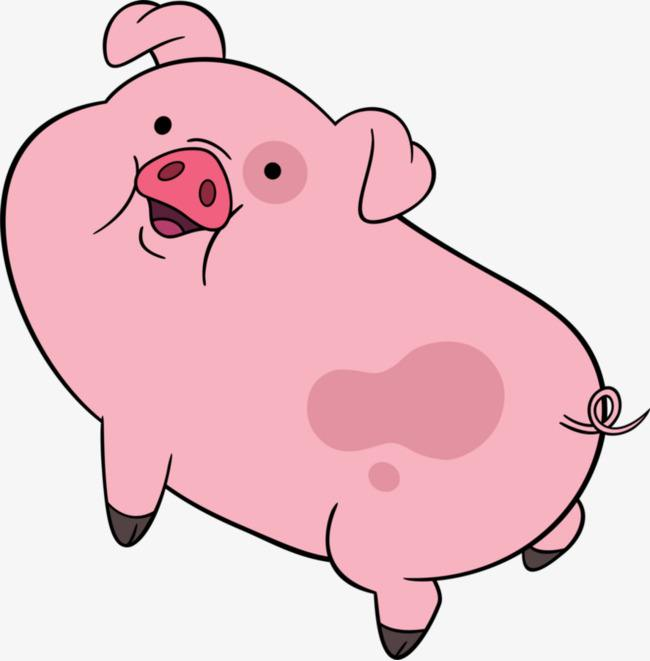# Aggressive cows

+关注继续查看

Farmer John has built a new long barn, with N (2 <= N <= 100,000) stalls. The stalls are located along a straight line at positions x1,...,xN (0 <= xi <= 1,000,000,000).

His C (2 <= C <= N) cows don't like this barn layout and become aggressive towards each other once put into a stall. To prevent the cows from hurting each other, FJ want to assign the cows to the stalls, such that the minimum distance between any two of them is as large as possible. What is the largest minimum distance?

* Line 1: Two space-separated integers: N and C

* Lines 2..N+1: Line i+1 contains an integer stall location, xi

* Line 1: One integer: the largest minimum distance

5 3
1
2
8
4
9

3

OUTPUT DETAILS:

FJ can put his 3 cows in the stalls at positions 1, 4 and 8, resulting in a minimum distance of 3.

Huge input data,scanf is recommended.

USACO 2005 February Gold

（参考http://blog.csdn.net/wuxiushu/article/details/49158843）

 1 #include<iostream>
2 #include<algorithm>
3 #include<stdio.h>
4 #include<string.h>
5 using namespace std;
6 const int MAX = 100010;
7 int a[MAX],n,m;
8
9 bool C(int d)
10 {
11     int t = a,count = 1;
12     for(int i = 1;i < n;i ++)
13     {
14         if(a[i] - t >= d)
15         {
16             count ++;
17             t=a[i];
18             if(count >= m)
19                 return true;
20         }
21     }
22     return false;
23 }
24
25
26 int solve()
27 {
28     int x = 0,y = a[n-1] - a;
29     while(x <= y)
30     {
31         int mid=(x+y)/2;
32         if(C(mid))
33             x=mid + 1;
34         else
35             y=mid - 1;
36     }
37     return x - 1;
38 }
39
40
41 int main()
42 {
43     while(~scanf("%d%d",&n,&m))
44     {
45         for(int i = 0;i < n;i ++)
46         scanf("%d",&a[i]);
47         sort(a,a+n);
48         printf("%d\n",solve());
49     }
50     return 0;
51 }63561 015896 0Xshell使用SSH远程登录阿里云ECS服务器CentOS7
21958 014878 015762 019077 036371 020273 023976 028299 0

543

0

JS零基础入门教程（上册）